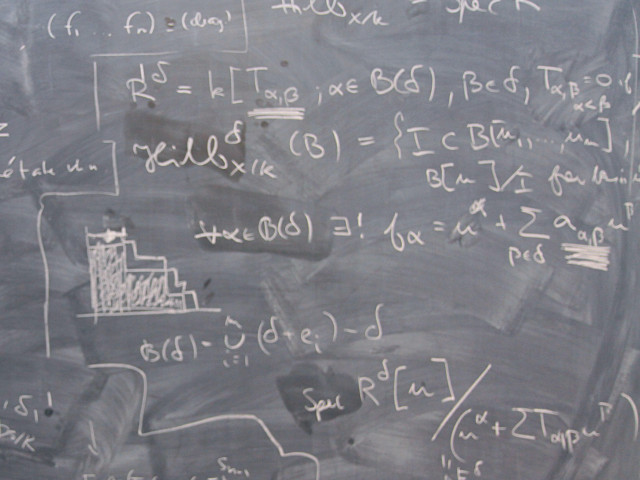# SF1631 Discrete Mathematics 12.0 credits

#### Diskret matematikBasic course of discrete mathematics and algebra.

### Offering and execution

Course offering missing for current semester as well as for previous and coming semesters

## Course information

### Content and learning outcomes

#### Course contents *

Bijections, injections, surjections. Principles of counting, Pascal´s triangle. Linear recursion. Partitions, equivalence relations. Modular arithmetic. Chryptography. Boolean algebra. Graph theory. Basic group theory. Chinese remainder theorem. Rings, fields, polynomials. Finite fields. Error correcting codes. Deeper study of some subject in graph theory.

#### Intended learning outcomes *

The general objective is to give basic knowledge in Discrete Mathematics, especially in the solution of combinatorial problems, the knowledge of some important algebraic structures and basic knowledge of graph theory. Also the ability to perform a stringent mathematical discussion is trained. More precisely after a course is expected to a students to:

• be a master of the application of the following combinatorial principles: Rule of multiplication, rule of addition, principle of inclusion exclusion, binomial coefficients, multinomial coefficients, Stirling numbers of the second kind.
• be able to, in some simple and obvious situations, to use the pigeon holeprinciple.
• be a master of the solution of linear recurrence equations with constant coefficients and the knowledge of the so called Master Theorem.
• be a master of the concepts injective, surjective and bijective functions.
• be a master of the description and the calculation of permutations.
• have some knowledge of infinite sets, especially the difference between countable and non countable infinite sets.
• be a master of equivalence relations and equivalence classes.
• be a master of the use of the algorithm of Euclid for the calculation of the greatest common divisor D and the solution of the Diophantine equation ax+by=D.
• have some elementary knowledge of prime numbers, the factorization into prime numbers and the fundamental theorem of aritmetic.
• be a master of the congruence calculus module a positive integer especially the rings Z/nZ and to be able to use the chines reminder theorem to perform calculations in those rings.
• be a master of the application of Fermat's Theorem and Euler's Theorem on congruences and to have an understanding of the Euler fi-function.
• be a master of the mathematical part of the RSA crypto.
• be a master of the application of the definitions of the concepts groups, rings and fields.
• be a master of the theorem of Lagrange for groups, subgroups, cosets of subgroups, the order of an element.
• be a master of cyclic groups and the symmetrical group.
• be a master of the application of the theorem of Burnside.
• know some elementary concepts from the theory of rings such as zero divisor, division rings and fields.
• be a master of the calculation in polynomial rings, especially such rings with coefficients in a field.
• be a master of the construction and calculation in finite fields.
• be a master of the construction of error correcting codes by the use of a parity-check matrix and a master of the concepts minimum distance and linear codes.
• be a master of elementary concepts from the theory of graphs as isomorphism, valence, connected graph, path, cycle, Hamiltonian graph, Eulerian graph.
• have some knowledge about the structure and elementary concepts and results of trees.
• have knowledge about different coloring problems for graphs.
• be a master of planar graphs, including the Euler formula and the theorem of Kuratowski.
• be a master of marriage theorem of Hall and the concepts maximal matching and alternating path.

Further, the students are forced to produce a small thesis with 2000-3000 words. This thesis will be the result of a study of a special field in Discrete Mathematics, e.g. graph theory. In connection with this study a realistic problem shall be formulated and solved. The thesis shall have a good linguistic presentation, a good layout and contain a good mathematical reasoning.

Finally, and as a consequence of the mathematics that have been studied, have achieved a better understanding for mathematics in general and an understanding for the advantage with a mathematical way to structure the solution of a problem.

#### Course Disposition

No information inserted

### Literature and preparations

#### Specific prerequisites *

SF1604 Linear algebra II (or equivalent).

#### Recommended prerequisites

No information inserted

#### Equipment

No information inserted

#### Literature

Biggs: Discrete Mathematics, 2:nd ed.

### Examination and completion

A, B, C, D, E, FX, F

#### Examination *

• LAB1 - Essay, 3.0 credits, Grading scale: A, B, C, D, E, FX, F
• TENA - Examination, 6.0 credits, Grading scale: A, B, C, D, E, FX, F
• TENB - Examination, 3.0 credits, Grading scale: A, B, C, D, E, FX, F

Based on recommendation from KTH’s coordinator for disabilities, the examiner will decide how to adapt an examination for students with documented disability.

The examiner may apply another examination format when re-examining individual students.

#### Other requirements for final grade *

Two written exams (TENA; 6 credits), (TENB; 3 cr).
One written essay (LAB1; 3 credits).
Optional home assignments can give extra points at the exam.
The final grade is decided by the grades of both parts of the examination.

#### Opportunity to complete the requirements via supplementary examination

No information inserted

#### Opportunity to raise an approved grade via renewed examination

No information inserted

### Further information

#### Course web

Further information about the course can be found on the Course web at the link below. Information on the Course web will later be moved to this site.

Course web SF1631

SCI/Mathematics

#### Main field of study *

Mathematics, Technology

First cycle